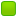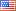###Author Topic: variables program  (Read 3108 times)

####fran2884

• New Member
• Posts: 1##### variables program
« on: June 19, 2014, 05:41:32 PM »

hi, I need to convert this function in c in assembly:
#define MATRIX float*
MATRIX prodMatrixRowCacheUnroll(MATRIX A, MATRIX B, int righeA, int colonneB, int RC, int block_size){

MATRIX Prod = alloc_matrix(righeA,colonneB);
int i,j,k,ii,jj,kk;
int unroll=10;
for (i=0;i<righeA;i+=block_size) {
for (j=0;j<colonneB;j+=block_size) {
for(k=0;k<RC;k+=block_size){
for (ii=i; ii<i+block_size; ii++) {
for (jj=j; jj<j+block_size; jj++){
for (kk=k; kk<k+block_size; kk+=unroll) {

Prod[(ii*colonneB)+jj]+=A[(ii*RC)+kk]*B[(kk*colonneB)+jj];
Prod[(ii*colonneB)+jj]+=A[(ii*RC)+(kk+1)]*B[((kk+1)*colonneB)+jj];
Prod[(ii*colonneB)+jj]+=A[(ii*RC)+(kk+2)]*B[((kk+2)*colonneB)+jj];
Prod[(ii*colonneB)+jj]+=A[(ii*RC)+(kk+3)]*B[((kk+3)*colonneB)+jj];
Prod[(ii*colonneB)+jj]+=A[(ii*RC)+(kk+4)]*B[((kk+4)*colonneB)+jj];
Prod[(ii*colonneB)+jj]+=A[(ii*RC)+(kk+5)]*B[((kk+5)*colonneB)+jj];
Prod[(ii*colonneB)+jj]+=A[(ii*RC)+(kk+6)]*B[((kk+6)*colonneB)+jj];
Prod[(ii*colonneB)+jj]+=A[(ii*RC)+(kk+7)]*B[((kk+7)*colonneB)+jj];
Prod[(ii*colonneB)+jj]+=A[(ii*RC)+(kk+8)]*B[((kk+8)*colonneB)+jj];
Prod[(ii*colonneB)+jj]+=A[(ii*RC)+(kk+9)]*B[((kk+9)*colonneB)+jj];
}
}
}
}
}
}
return Prod;
}

is a product of matrices, where A is the transpose of B, with unrolling techniques and cache blocking, I'm new assembly nasm, and I began to write this function:

%include "sseutils.nasm"

section .data
section .bss
section .text

global main

A equ 8
beta equ 12
m equ 16 ; matrix rows
n equ 20 ; matrix columns

main:
push ebp ; Base Pointer
mov ebp, esp ; il Base Pointer point to current record activation
push ebx
push esi
push edi

mov eax, [ebp+A] ; address of MATRIX A
mov ebx, [ebp+beta] ; address of beta
mov ecx, [ebp+m] ; m rows
mov edx, [ebp+n] ; n columns

call prodMatrixRowCacheUnroll

....

call prodMatrixRowCacheUnroll:

.....

the point is that since I have to do it with the X86 architecture, as I memorize all the variables i, ii, j, jj, BLOCK_SIZE, k, kk? there are not enough registers, maybe it will be a trivial question, but I'm newee and do not know how to do, I have to translate this function and are in trouble because i have
very short time .. thanks

####Frank Kotler

• NASM Developer
• Hero Member
•• Posts: 2436
• Country:##### Re: variables program
« Reply #1 on: June 19, 2014, 10:25:05 PM »
No offense intended, but if you're new to this - or even fairly new - you've bitten off more than you can chew. You'll only frustrate yourself. I strongly suggest you start with something simpler - much simpler - and work up to this in small steps. If you're short on time, yes, you're in trouble. Ask for an extension. Better yet, ask for an assignment more suitable for a beginner.

Having said that, you can get your compiler to spit out the assembly code it's using. For gcc, the switch is "-S". For a Microsoft product, I think the switch is "/Fa"... but that was a long time ago. Other compiler switches will make a difference, too. If you don't ask for optimization, you'll get fairly dumb code. If you ask for optimization, you'll get better code but it will probably be harder to understand. Try it both ways, perhaps. In any case, it will not be suitable to assemble with Nasm, but may serve as a guide to what you want to do.

I wish you all the luck in the world - you're going to need it!

Best,
Frank

####gammac

• Jr. Member
•• Posts: 71
• Country:##### Re: variables program
« Reply #2 on: June 20, 2014, 07:09:04 AM »

... the point is that since I have to do it with the X86 architecture, as I memorize all the variables i, ii, j, jj, BLOCK_SIZE, k, kk? there are not enough registers, maybe it will be a trivial question, but I'm newee and do not know how to do, ...

I agree with Frank Kotler but inside a function or procedure you can hold local variables on the stack. e.g.:

Code: [Select]
`cpu 386[section .code use32]my_func: push ebp mov ebp, esp sub esp, 8      ; here you made space for 2 dword vars        mov   [ebp-4], 1   ; initialize the 1st var with number one        mov   [ebp-8], 10  ; initialize the 2nd var with number ten mov esp, ebp pop ebpret`